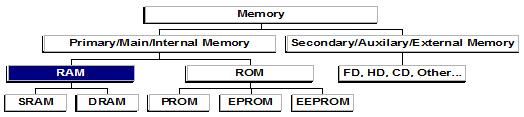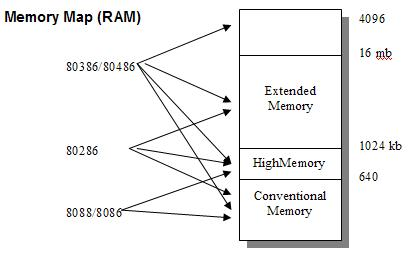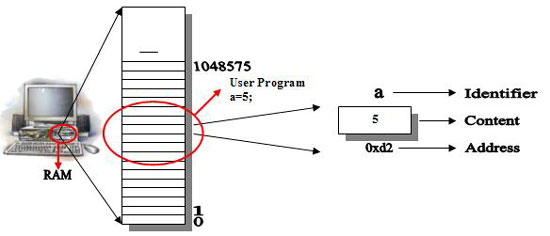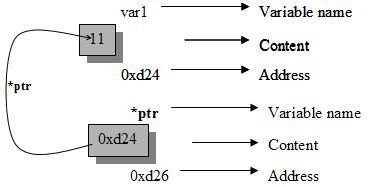# Learning C/C++ Step-By-Step - Page 9

### 09. Step-by-Step C/C++ --- C Programming - Pointers

#### Pointers

 1. About Memory 2. Addressing Scheme 3. How to find the address of a Variable 4. Pointers 5. Pointer Arithmetic 6. Pointers and Arrays 7. Pointers and Strings 8. Glossary

Computer has the feature to store data, and manipulate them. Storage of data requires a storage device, which was comfortable to store and retrieve data quickly and accurately with out confusion. Commonly Computer has to compromise with two storage methods.SRAM               Static Random Access Memory
DRAM               Dynamic Random Access Memory
EEPROM          Electrically Erasable Programmable Read Only Memory

Memory chips can store data, instructions and intermediate & final results. The memory is organized into bytes, each byte capable of storing one character of information. Each byte of memory has an address or location number, which uniquely identifies it. The size of memory is measured either in kilobytes (KB), megabytes (Mb), gigabytes or terabytes (TB).

RAM:
Memory device is a storage location to store information.
The Vital Computer resource memory is allocated to each of the variable after their declaration in the C-Program. The type of the variable decides the number of bytes of memory to be allocated to each variable.The above picture tells you the following information.

 1. RAM is a temporary memory and a part of the computer. 2. It can hold the value of program. 3. Every byte in RAM has identified with a unique positive number called address. 4. Addresses are as numbers, just as they are for houses on a street. 5. The number starts at 0 and go up from there 1-2-3 and so on. 6. If we have 640 KB of memory the highest address is 655, 359, for 1mb of memory it is 1,048,575. 7. Our program, when it is loaded into memory, occupies a certain range of these addresses. 8. That means that every variable and every function in out program starts at a particular address.

#### 3. How to find the address of a Variable

In the last section point 8 tells each and every variable/function starts at a particular address. Addresses are unique positive numbers in the hexa decimal format.

& ( address of ) - It can tell you the address of variable / function in the current program.

The following program demonistrates to find the address of a variable ‘a’

 /* 58_address.c */ #include int main() {      int a = 10;      printf("\n Value of A is : %d", a);      printf("\n Address of A is : %d", &a);      return 0; }

Replace the above marked format values with the following format to get absolute hexadecimal address value.
0x%x
Ex.
` printf("\nAddress of A is     : 0x%x", &a);`

Program to find the address of function ‘disp()’

 /* 59_address.c */ #include void disp() {      printf("\nHello");      printf("\nHow are");      printf("\nYou"); } int main() {      disp();      printf("\nAddress of disp() : 0x%x", &disp);      return 0; }

#### 4. Pointers

According to the last section we know how to find and display the address of a variable/function.
This time we learn about how to store the address of a variable/function in another variable.

Note: Variables can hold constant values.

 Try with the following: int a, b; a = 5;            /* Valid */ b = &a;           /* In valid */ Again Try with the following: int a, *b; a = 5;            /* Valid */ b = &a;           /* Valid */

b = &a; correct! Yes, variables (General variables) are unable to hold addresses. But variables proceeded with ‘*’ (Pointer Variables) are able to hold both constant values as well as address of another variables/functions.

Pointer: Variable that holds address values.

 Variables (General) General variable performs only one operation to hold constant values Pointer variables ( Variables preceded with ‘*’) Pointer variables can perform two operations to hold constant values as well as address values of other variables/functions

Reference to / Pointer to / Content at address (*)

`int *ptr;`

To the uninitiated this is a rather bizarre syntax. The asterisk means pointer to. Thus the statement defines the variable ptr as a pointer to int. This is another way of saying that the variable can hold the address of integer variables.

 If we called it, type pointer we could write declaration like. `pointer ptr;           /* invalid */` The problem is that the compiler need to know what kind of variable the pointer points to.
 Declaration of a pointer variable char *cptr;           /* Pointer to character */ int *iptr;            /* Pointer to int */ float *fptr;            /* Pointer to float */ struct emp *e;            /* Pointer to abstracted data emp e */

#### Accessing the variable Pointed to:

Here is the special way to access the values of a variable using its address instead of its name.
 /* 60_addr.c */ #include int main() {      int var1 = 11;           /* variable var1 = 11 */      int *ptr;           /* Variable ptr as pointer to */      ptr = &var;           /* Hold the address of var to ptr */      printf("Value of var1 is %d", *ptr);           /* Pointer to the address of var1 */      return 0; }If the statement is printf (“%d”, ptr); then it displays the value of ptr means the address of var1, but the above statement can display the content of the address, which was stored in variable ptr.

Program to demonstrate the use of Address_Of and Pointer_To

 /* 61_ptrdemo.c */ #include int main() {      int a = 10, *p;           /* Integer a and pointer p */      p = &a;           /* Assign address of a to p */      printf("\nValue of A : %d", a);           /* Content of a */      printf("\nAddress of A : 0x%x", &a);           /* Address of a */      printf("\nValue of P : 0x%x", p);           /* Content of p */      printf("\nAddress of P : 0x%x", &p);           /* Address of p */      printf("\nContent at address of a : %d", *p);           /* Pointer to &a */      return 0; }

#### 5. Pointer Arithmetic

All the variables can support arithmetic operations, as well as we can perform arithmetic operation on pointers also. C/C++ language can supports 4 Arithmetic operations on Pointers namely.

 Operation Addition Subtraction Incrementation Decrementation Symbol + - ++ --

Note: The main characteristic of pointer arithmetic is that the above operators in bytes with reference to its variable type.
 /* 62_ptr.c */ /* Demonistration of pointer arithmetic */ #include int main() {      int a, *p;      a = 100;      p = &a;      (*p)++; /* Increment pointer to (content at address) by 1 */      printf("%d", *p);      return 0; } Output 101

Demonstration of Pointer arithmetic, Increment the address value

 /* 63_ptr.c */ /* Increment the address value by 1 */ #include int main() {      int a, *p;      a = 100;      p = &a;      *p++; /* Increment the address value in p by 1 */      printf("%d", *p);      return 0; } Output Unexpected output

The above program illustrates the arithmetic operators with respective of both value and address incrementation. p is a pointer variable and a is assigned with 100, as well as p is assigned with the address of a.

Now *p++ effects incrementing or actually skipping the memory by 2 bytes to get new address and their its content.

If it’s (*p)++, then that the content pointed by p is 100 is incremented, resulting 101.

#### 6. Pointers and Arrays

In C/C++ language the data types pointers and arrays resembles with each other. The array element references as well as the pointer variable, both are used to hold the address of data elements in memory.

 char name; Or char *name ; char months; Or char **months;

There is a close association between pointers and arrays. Here is a review on arrays.

 /* 64_ptrarr.c */ #include int main() {      int i, a = { 56, 43, 78, 98, 12 };      for( i = 0, i < 5; i++)           printf("\n%d", a[i]);      return 0; }

There is a possibility to access array elements using pointer notation.
Find the output of the following program.

 /* 65_ptrarr.c */ #include int main() {      int i, a = { 56, 43, 78, 98, 12 };      for( i = 0, i < 5; i++)           printf("\n%d", *(a+ i) );      return 0; }

 /* 66_ptrarr.c */ #include int main() {      int i, a[ ] = { 56, 43, 78, 98, 12 }, *p;      p = a;      for( i = 0, i < 5; i++)           printf("\n%d", *(p+ i) );      return 0; }

Here is an easiest approach to print the elements of the given array (size not required).

 /* 67_ptrarr.c */ #include int main() {      int i, a[ ] = { 56, 43, 78, 98, 12 }, *p;      p = a;      while (*p)           /* or for(int i = 0; i<5; i++ ) */           printf("\n%d", *p++);      return 0; }

#### 7. Pointers and Strings

A string is a collection of characters including spaces. This time we discuss about how to handle strings using pointers. No more discussions to make confusion. Here is the simple task to verify both pointer and array of strings.

There is a subtle difference between strings & pointers follow the program.

 /* 68_ptrstr.c */ #include int main() {      char str1[ ] = "You would like to explore C.";      char *str2 = "You would like to explore C.";      puts(str1);      puts(str2);      str1++; /* Invalid expression */      str2++; /* Valid expression */      puts(str2); /* prints ou would like to…… */      return 0; }

Strings as Function Arguments

A pointer variable is more flexible than array variables, Here is the program to demonstrate & displays a string with pointer notation.

 /* 69_ptrarr.c */ #include void disp(char *p); int main() {      char str[ ] = "Hello!!..Hello!!.. Pointers can handle it?";      disp(str);      return 0; } void disp(char *p) {      while(*p)      printf("%c", *p++); }

Array of pointers to strings

There is a disadvantage to store an array of strings, in that the sub arrays that hold the string must all be the same length. So that space is wasted when strings are shorter than the sub arrays.
Here is the solution:

 /* 70_strings.c */ #include int main() {      char *weeks[7 ] = { "Sunday", "Monday", "Tuesday", "Wednesday", "Thursday", "Friday", "Saturday" };      int i;      for( i = 0; i<7; i++)           puts(weeks[ i ] );      return 0; }

When strings are not part of an array, C/C++ places them contiguously in memory, So there is no wasted spaces.

/* An example program to hold an array of pointers of type ‘int’ */

 /* 71_ptrarr.c */ #include int main() {      int *arr; /* Array of int pointers */      int i = 31, j = 5, k = 19, l = 71, m;      arr = &i;      arr = &j;      arr = &k;      arr = &l;      for(m = 0; m <= 3; m++)           printf("\n%d", *(arr[m]) );      return 0; }

A look on Library Functions

We are already familiar with standard string functions. They have string arguments that are specified using pointer notation,
If we are clear with pointers & strings concept, we are able to write our own string functions.
Here is an example program to copy string.

/* Copies one string to another with pointers */

 /* 72_strcpy1.c */ #include void strcpy1(char * dest, char *src); int main() {      char *str1 = "How can I learn more about C/C++ !!!";      char *str2;      strcpy1(str2, str1);      puts(str2);      return 0; } void strcpy1(char * dest, char *src) {      while(*src)           *dest++ = *src++;      *dest = ‘\0’; }

#### 8. Glossary

 Address A value that points to a location in memory. A pointer contains the address or location of a value, as opposed to the value itself. Array An array is a collection of data items of the same type. Contiguous A storage characteristic that specifies that the values are stored in consecutive locations either in memory or on disk. Function A series of instructions to perform a specific task, which can be combined with other functions to create a program. Memory Descriptive of a device or medium that can accept data, holds them, and deliver them on demand at a later time. Synonymous with storage. Pointer Contains the address or memory location of a value, as opposed to the value itself. RAM (Random Access Memory) 1. A storage device structured so that the time required retrieving data is not significantly affected by the physical location of the data.  2. The primary storage section of a personal computer. String An array capable of storing zero or more characters. In C. a string is declared as a character array with the NULL (\0) character appended to specify the end of the string. Variable A name associated with a location in memory whose value can change during program execution.view as pdf |print